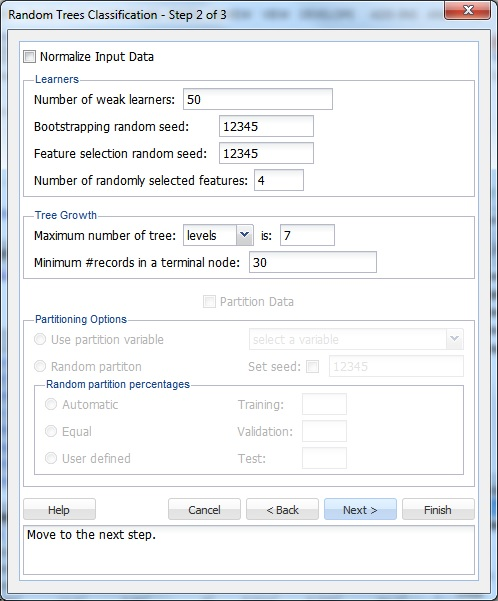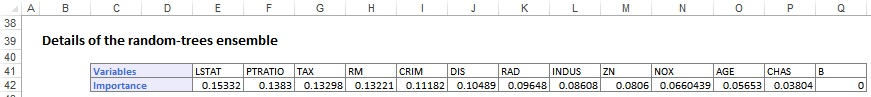This example illustrates the Classification - Random Trees Ensemble Method using the Boston_Housing.xlsx data set, and compares the results of all three ensemble methods.

On the XLMiner ribbon, from the Data Mining tab, select Classify - Classification Tree - Random Trees to open the Random Trees Classification - Step 1 of 3 dialog. Select a cell on the the Data_Partition worksheet.At Output Variable, select CAT. MEDV, then from the Selected Variables list, select all remaining variables except MEDV. (The MEDV variable is not included in the Input, as it is not a categorical variable.) The CAT. MEDV variable is a categorical variable that is based on the MEDV variable.

Choose the value that will be the indicator of Success by clicking the down arrow next to Specify “Success” class (for Lift Chart). In this example, we will use the default of 1.

At Specify initial cutoff probability for success, enter a value between 0 and 1 for. If the Probability of success (probability of the output variable = 1) is less than this value, then a 0 is entered for the class value, otherwise a 1 is entered for the class value. In this example, we will keep the default of 0.5.

Click Next to advance to the Random Trees Classification - Step 2 of 3 dialog.When Normalize Input Data is selected, XLMiner normalizes the data to determine if linear combinations of the input variables are used when partitioning the tree. Keep this option unchecked.

Leave the Number of weak learners at the default of 50. This option controls the number of weak classification models that are created. The ensemble method stops when the number or classification models created reaches the value set for the Number of weak learners. The algorithm then computes the weighted sum of votes for each class and assigns the winning classification to each record.

For Bootstrapping random seed and Feature selection random seed, leave the default of 12345. These values set the random seed that results in the same observations being chosen for the Training/Validation/Test Sets each time a standard partition is created.

The Random Trees ensemble method works by training multiple weak classification trees using a fixed number of randomly selected features, then taking the mode of each class to create a strong classifier. The Number of randomly selected features controls the fixed number of randomly selected features in the algorithm. Leave this option at the default setting of 4.

Under Tree Growth, leave the defaults of levels and 7 for Maximum number of tree. The tree may also be limited by the number of splits or nodes by clicking the drop-down next to levels. If levels is chosen, the tree contains the specified number of levels. If splits is selected, the number of times the tree is split is limited to the value entered, and if nodes is selected, the number of nodes in the entire tree is limited to the value specified.

Set Minimum # records in a terminal node to 30. XLMiner stops partitioning (splitting) the tree when all nodes contain a minimum of 30 records.

XLMiner V2015 provides the ability to partition a data set from within a classification or prediction method by selecting Partition Options on the Step 2 of 3 dialog. If this option is selected, XLMiner partitions the datadset (according to the partition options) immediately before running the prediction method. If partitioning has already occurred on the data set, this option is disabled. For more information on partitioning, see the Data Mining Partition section.

Click Next to advance to the Random Trees Classification - Step 3 of 3 dialog.Under both Score Training Data and Score Validation Data, Summary Report and Lift Charts are selected by default. Select Detailed Report under both Score Training Data and Score Validation Data to produce a detailed assessment of the performance of the tree in both sets. Since we did not create a test partition, the options for Score Test Data are disabled. See the Data Mining Partition section for information on how to create a test partition.

Click Finish to run the ensemble method. Worksheets containing the output of the Ensemble Methods algorithm are inserted at the end of the workbook. Click the CTRandTrees_Output worksheet to view the Output Navigator. Click any link in this section to navigate to various sections of the output.Click the Ensemble Details link to navigate to the Details of the random-trees ensemble table. This table displays the variables that are included in the model along with their importance.

Scroll down the CTRandTrees_Output worksheet to view the Details of the boosting tree ensemble. The importance percentage for each variable is listed here, and measures the variable's contribution in reducing the total misclassification error.Scroll down to Training Data Scoring - Summary Report to view the Confusion Matrix.The Confusion Matrix displays counts for cases that were correctly and incorrectly classified in the Training and Validation Sets. Six records were misclassified in the Training Set, resulting in an error of 1.97%, and six records were misclassified in the Validation Set, resulting in a % error of 2.97%.

Click the CTRandTrees_TrainScore tab to view the Predicted Class, Actual Class, Probability for 0, and Probability for 1 (success) values in the Training Set. If the value for Probability for 1 is greater than 0.5, the record is assigned a classification of 1.The same applies to the CTRandTrees_ValidScore tab.Click the CTRandTrees_TrainLiftChart and CTRandTrees_ValidLiftChart tabs to navigate to the Lift Charts, shown below.

Lift Charts consist of a lift curve and a baseline. After the model is built using the Training Set, the model is used to score on the Training and the Validation Sets (if one exists). Then the data set(s) are sorted using the predicted output variable value. After sorting, the actual outcome values of the output variable are cumulated, and the lift curve is drawn as the number of cases (X-axis) versus the cumulated value (Y-axis). The baseline (red line connecting the origin to the end point of the blue line) is drawn as the number of cases versus the average of actual output variable values multiplied by the number of cases. The greater the area between the lift curve and the baseline, the better the model.

In the Lift Chart (Training Set) pictured below, the red line originating from the origin and connecting to the point (300, 47) is a reference line that represents the expected number of CAT MEDV predictions if XLMiner simply selected random cases  (i.e., no model was used). This reference line provides a yardstick against which to compare the model performance. From the Lift Chart below, we can infer that if we assigned 200 cases to class 1, about 47 1s would be included. If 200 cases were selected at random, we could expect about 30 1s.

The decile-wise lift curve is drawn as the decile number versus the cumulative actual output variable value divided by the decile's mean output variable value. The bars in this chart indicate the factor by which the MLR model outperforms a random assignment, one decile at a time. Refer to the validation graph below. In the first decile, taking the most expensive predicted housing prices in the data set, the predictive performance of the model is about 6.5 times better as simply assigning a random predicted value.

ROC curves plot the performance of binary classifiers by graphing true positive rates (TPR) versus false positive rates (FPR), as the cutoff value grows from 0 to 1. The closer the curve is to the top left corner of the graph (the smaller the area above the curve), the better the performance of the model.

In an ROC curve, we can compare the performance of a classifier with that of a random guess, which would lie at a point along a diagonal line (red line) running from the origin (0, 0) to the point (1, 1). (This line is sometimes called the line of no-discrimination.) Anything to the left of this line signifies a better prediction and anything to the right signifies a worse prediction. The best possible prediction performance would be denoted by a point at the top left of the graph at the intersection of the x and y axis. This point is sometimes referred to as the perfect classification. Area Under the Curve (AUC) is the space in the graph that appears below the ROC curve. (This value is reported at the top of the ROC graph.) AUC is a value between 0 and 1. The closer the value AUC is to 1, the better the performance of the classification model. In this example, the AUC equals 0.994743 in the training set which means that XLMiner achieved almost perfect classification in the Training Set. In the Validation Set ROC, the AUC is also very close to 1 (AUC = .995659), which indicates that this model is a very good fit to the Validation Set as well.Since the number of trees produced when using an Ensemble Method can potentially be in the hundreds, it is not practical for XLMiner to draw each tree in the output.

XLMiner generates the CTRandTrees_Stored worksheet along with the other output sheets. For information on scoring new data, see the Scoring New Data section.